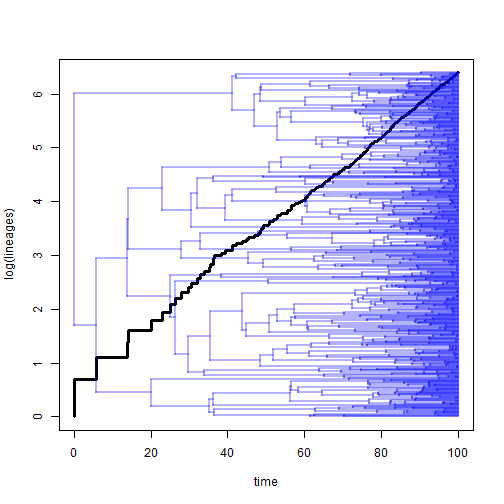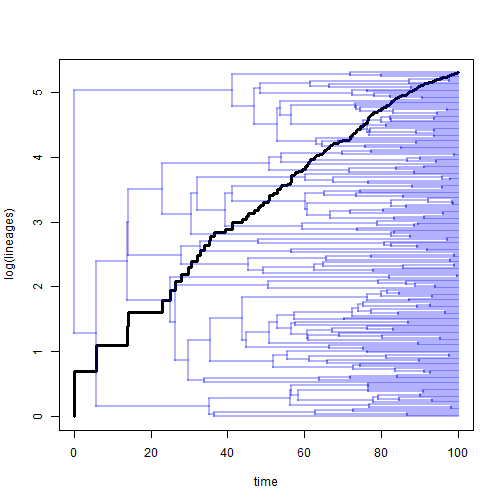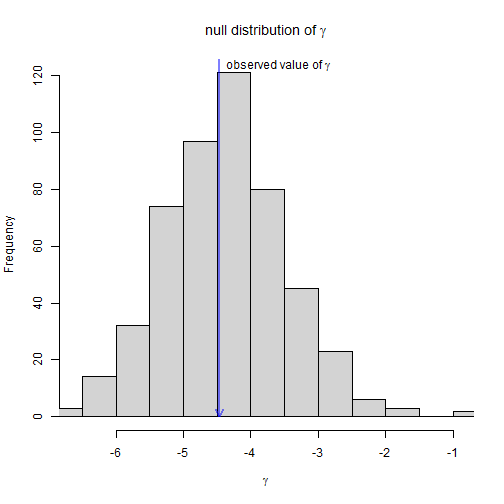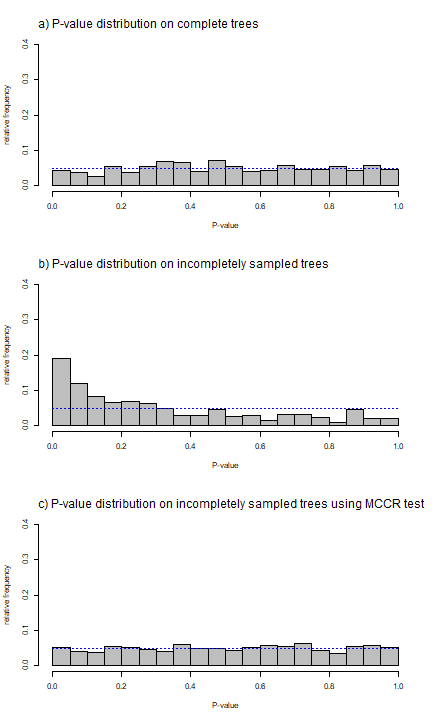## Saturday, January 19, 2019

### MCCR test now in phytools

Yesterday I posted about implementing Pybus & Harvey's (2000) MCCR test. This used to be in Dan Rabosky's laser, which is no longer available (except in archived versions) on CRAN. The method is quite simple as it merely uses Monte Carlo simulation to generate a null distribution of the γ-statistic for incompletely sampled phylogenies. I have now added this function (and a couple of associated methods) to phytools. This update can be obtained by installing the latest version of phytools from GitHub using devtools.

Here's a quick demo of how the function works:

``````library(phytools)
packageVersion("phytools")
``````
``````##  '0.6.66'
``````
``````tree<-pbtree(n=600,scale=100)
ltt(tree,show.tree=TRUE,lwd=3)
````````````## Object of class "ltt" containing:
##
## (1) A phylogenetic tree with 600 tips and 599 internal nodes.
##
## (2) Vectors containing the number of lineages (ltt) and branching times (times) on the tree.
##
## (3) A value for Pybus & Harvey's "gamma" statistic of -0.1158, p-value = 0.9078.
``````
``````## subsample the tree to introduce a downward bias in gamma:
incomplete<-drop.tip(tree,sample(tree\$tip.label,400))
ltt(incomplete,show.tree=TRUE,lwd=3)
````````````## Object of class "ltt" containing:
##
## (1) A phylogenetic tree with 200 tips and 199 internal nodes.
##
## (2) Vectors containing the number of lineages (ltt) and branching times (times) on the tree.
##
## (3) A value for Pybus & Harvey's "gamma" statistic of -4.4687, p-value = 0.
``````
``````## MCCR test to take into account incomplete sampling:
object<-ltt(incomplete,plot=FALSE)
mccr.result<-mccr(object,rho=200/600,nsim=500)
mccr.result
``````
``````## Object of class "mccr" consisting of:
##
## (1) A value for Pybus & Harvey's "gamma" statistic of -4.4687.
##
## (2) A two-tailed p-value from the MCCR test of 0.92.
##
## (3) A simulated null-distribution of gamma from 500 simulations.
``````
``````plot(mccr.result)
``````We can also do a small experiment to show that the MCCR test has the correct type I error rate. Here I'm going to simulate five hundred 150 taxon trees, each of which I proceed to randomly subsample to include only 100 taxa. I'll then compute γ & a P-value for the null hypothesis test of γ equal to zero on the original tree; the same things on the subsampled tree; and finally a P-value based on the MCCR method:

``````foo<-function(){
full<-pbtree(n=150)
full.ltt<-ltt(full,plot=FALSE)
incomplete<-drop.tip(full,sample(full\$tip.label,50))
incomplete.ltt<-ltt(incomplete,plot=FALSE)
setNames(c(full.ltt\$gamma,full.ltt\$p,
incomplete.ltt\$gamma,incomplete.ltt\$p,
mccr(incomplete.ltt,rho=100/150,nsim=200)\$'P(two-tailed)'),
c("full-gamma","full-P(gamma)","inc-gamma",
"inc-P(gamma)","P(mccr)"))
}
sim.Result<-t(replicate(500,foo()))
bb<-seq(0,1,by=0.05)
par(mfrow=c(3,1))
obj<-hist(sim.Result[,2],bb,plot=FALSE)
obj\$counts<-obj\$counts/nrow(sim.Result)
plot(obj,ylab="relative frequency",col="grey",ylim=c(0,0.4),xlab="P-value",main="")
lines(c(0,1),rep(0.05,2),col="blue",lty="dotted")
mtext(text="a) P-value distribution on complete trees",adj=0,line=1,
cex=1)
obj<-hist(sim.Result[,4],bb,plot=FALSE)
obj\$counts<-obj\$counts/nrow(sim.Result)
plot(obj,ylab="relative frequency",col="grey",ylim=c(0,0.4),xlab="P-value",main="")
lines(c(0,1),rep(0.05,2),col="blue",lty="dotted")
mtext(text="b) P-value distribution on incompletely sampled trees",adj=0,line=1,
cex=1)
obj<-hist(sim.Result[,5],bb,plot=FALSE)
obj\$counts<-obj\$counts/nrow(sim.Result)
plot(obj,ylab="relative frequency",col="grey",ylim=c(0,0.4),xlab="P-value",main="")
lines(c(0,1),rep(0.05,2),col="blue",lty="dotted")
mtext(text="c) P-value distribution on incompletely sampled trees using MCCR test",
``````We can see that the parametric test has good type I error on completely sampled tree, but elevated error on incompletely sampled trees. The MCCR test recovers this good type I error of the parametric test when our taxon sampling is incomplete. We can also compute the type I errors directly as follows:

``````typeI<-setNames(c(mean(sim.Result[,2]<=0.05),
mean(sim.Result[,4]<=0.05),mean(sim.Result[,5]<=0.05)),
c("complete","incomplete","MCCR"))
typeI
``````
``````##   complete incomplete       MCCR
##      0.044      0.190      0.052
``````

Neat.

#### 1 comment:

1.Hi Liam,

is there any chance to include the results of the MCCR test in the LTT plot as seen here (https://link.springer.com/article/10.1186/s12862-015-0435-9/figures/2)?

Cheers Josef

Note: due to the very large amount of spam, all comments are now automatically submitted for moderation.